# ISEE Upper Level Quantitative : How to find the missing part of a list

## Example Questions

### Example Question #161 : Data Analysis

Which is the greater quantity?

(a) The number of odd integerssuch that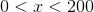(b) The number of even integerssuch that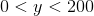(a) and (b) are equal.

(a) is greater.

It is impossible to tell from the information given.

(b) is greater.

(a) is greater.

Explanation:

This question can be most easily answered by matching each element in the set in (a) with the next consecutive integer, which is in the set in (b):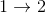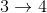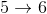...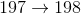Every element in the second set has a match, but there is an unmatched element in the first set. Therefore (a) is the greater quantity.

### Example Question #162 : Data Analysis

Which of the following is the greater quantity?

(a) The sum of the even integers fromto(b) Twice the sum of the integers fromto(a) is greater.

It is impossible to tell from the information given.

(a) and (b) are equal.

(b) is greater.

(a) and (b) are equal.

Explanation:

The quantities are equal. This can be proved as follows:

The sum of the integers fromtois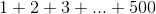.

(b) is twice this: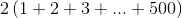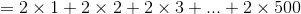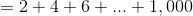This is the same value as (a), the sum of the even integers fromto.

### Example Question #11 : Sets

An arithmetic sequence begins as follows: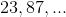Which is the greater quantity?

(a) The fourth term of the sequence

(b) 200

It is impossible to tell from the information given

(a) is greater

(b) is greater

(a) and (b) are equal

(a) is greater

Explanation:

The common difference of the sequence is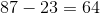, so the next two terms of the sequence are: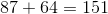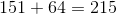215 is the fourth term. This makes (a) greater.

### Example Question #12 : Sets

A geometric sequence begins as follows: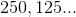Which is the greater quantity?

(a) The fourth element of the sequence

(b) 30

It is impossible to tell from the information given

(a) is greater

(a) and (b) are equal

(b) is greater

(a) is greater

Explanation:

The common ratio of the sequence is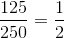The next two terms of the sequence can be found as follows: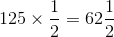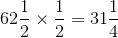This is the fourth term, which is greater than 30.

### Example Question #13 : Sets

A geometric sequence begins as follows: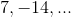Which is the greater quantity?

(a) The fourth term of the sequence

(b) The sixth term of the sequence

(a) and (b) are equal

(a) is greater

It is impossible to tell from the information given

(b) is greater

(a) is greater

Explanation:

The common ratio of the geometric sequence is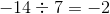The next four terms of the sequence are: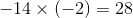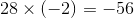- the fourth term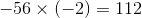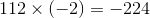- the sixth term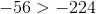, so the fourth term, which is (a), is greater

### Example Question #162 : Data Analysis

An arithmetic sequence begins as follows: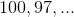Which of the following is the greater quantity?

(a) The tenth element of the sequence

(b) 70

(a) is greater

(b) is greater

It is impossible to tell from the information given

(a) and (b) are equal

(a) is greater

Explanation:

The common difference of the sequence is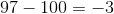; then tenth element is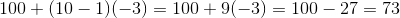,

which is greater than 70.

### Example Question #163 : Data Analysis

A standard deck of cards is altered by removing the red queens and the three of the jacks. A card is drawn at random from this altered deck.

Which is the greater quantity?

(a) The probability of drawing a red card

(b) The probability of drawing a black card

(b) is greater.

It is impossible to tell from the information given.

(a) is greater.

(a) and (b) are equal.

(b) is greater.

Explanation:

The three removed jacks must either comprise two red cards and one black card, or one red card and two black cards. Since the two red queens are also removed, the net result is either the removal of four red cards and one black card, or three red cards and two black cards. Either way, more black cards than red cards are left in the altered deck, and (b) must be greater.

### Example Question #171 : Data Analysis

An arithmetic sequence begins as follows: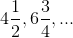'

Which is the greater quantity?

(a) The fifth number in the sequence

(b)(a) is greater.

(b) is greater.

It is impossible to tell from the information given.

(a) and (b) are equal.

(b) is greater.

Explanation:

The common difference of the sequence is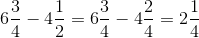.

The fifth number in the sequence is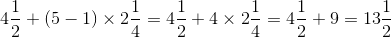.

This makes (b) greater.

### Example Question #171 : Data Analysis

A geometric sequence begins as follows: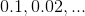Which is the greater quantity?

(a) The fifth term of the sequence

(b)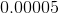(a) is greater.

(b) is greater.

(a) and (b) are equal.

It is impossible to tell from the information given.

(a) is greater.

Explanation:

The common ratio of the sequence is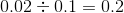.

The next three terms of the sequence are: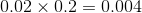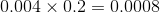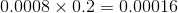, the fifth term.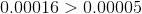, making (a) greater.

### Example Question #173 : Data Analysis

A geometric sequence begins as follows: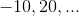Which is the greater quantity?

(a) The nine hundred ninety-ninth term of the sequence

(b) The one-thousandth term of the sequence

It is impossible to tell from the information given.

(b) is greater.

(a) is greater.

(a) and (b) are equal.

The common ratio is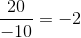, which means that the terms will alternate in sign. The first term is negative; therefore, all of the odd-numbered terms will be negative and all of the even-numbered terms will be positive. This makes the one thousandth term greater than the nine hundred ninety-ninth.